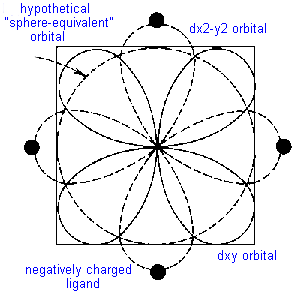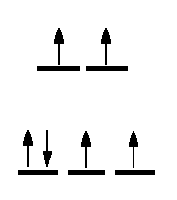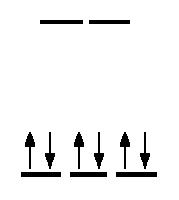Crystal Field Theory (CFT), An Introduction.

Lecture 3. CHEM1902 (C 10K) Coordination Chemistry

In the ionic CFT, it is assumed that the ions are simple point charges. When applied to alkali metal ions containing a symmetric sphere of charge, calculations of bond energies are generally quite successful. The approach taken uses classical potential energy equations that take into account the attractive and repulsive interactions between charged particles (that is, Coulomb's Law interactions).

The bond energy between the charges is proportional to q1 * q2/r

where q1 and q2 are the charges of the interacting ions and r is the distance separating them. This leads to the correct prediction that large cations of low charge, such as K+ and Na+, should form few coordination compounds.

For transition metal cations that contain varying numbers of d electrons in orbitals that are NOT spherically symmetric, however, the situation is quite different. The shape and occupation of these d-orbitals then becomes important in an accurate description of the bond energy and properties of the transition metal compound.

To be able to understand and use CFT then, it is essential to have a clear picture of the shapes (angular dependence functions) of the d-orbitals.

JSmol is an Open Source application for the display of molecular graphics that is capable of displaying atomic orbitals so it is possible to see the relationship between their orientation and ligands in different stereochemistries. See the cases for octahedral, tetrahedral and square planar complexes.

A cube provides a convenient reference for displaying the coordination centre of complexes since octahedral compounds have cubic symmetry i.e. the six anions sit at the centres of the faces of the cube and for tetrahedral complexes the 4 ligands sit at opposite diagonal edges.

You should be able to see that two of the d-orbitals, the dz2 and dx2-y2 meet the faces of the cube, but the remaining three (dxy, dyz and dxz) point towards the edges of the cube and actually have a node meeting the centres of the faces.

What happens then to the energy of the d-electrons if negatively charged ligands are brought in towards the metal centre to give an octahedral complex - where the ligands sit at the centre of the faces of the cube i.e. on the X, Y and Z axes? Since the d electrons are themselves negatively charged they would experience repulsion and their energies would be raised. For 's' and 'p' electrons this is of little consequence but for 'd' electrons there will be an energy difference involved depending on the orbital distribution and occupation.

Consider the simple example of TiCl63- in which six chloride ions octahedrally surround the Ti3+ cation. There is only one d-electron to be allocated to one of the five d- orbitals. If it were to occupy the dz2 or dx2-y2 orbital, both of which meet the face of the cube and thus point directly towards the chloride ligands, it would be strongly repelled. The geometry of these orbitals and their nodes would require the electron to stay near the negatively charged ligands causing even more repulsion than a spherically distributed electron would experience. On the other hand, if the electron were to occupy the dxy, dyz or dxz orbital, it would spend less time near the ligands than would a spherically distributed electron and would be repelled less.

This difference between for example the dx2-y2 orbital, the dxy orbital and a spherical distribution can be graphically represented byThe result of these differences for the d-orbitals is an energy difference between the dz2 and dx2-y2 orbitals compared to the dxy, dyz and dxz orbitals.The CFT approach can be easily extended to other geometries and the next most important case is the tetrahedron. To predict the splitting pattern of the energy of the d-orbitals under a tetrahedal crystal field you may once again find it convenient to consider how the ligands can fit into a cube to give a tetrahedron.

The next step is to consider how the d-orbitals interact with these incoming ligands. For tetrahedral complexes, the energy of those orbitals which point towards the edges should now be raised higher than those which point towards the faces. That is, the exact opposite of the situation we just dealt with for the octahedral crystal field. The end result is a splitting pattern which is represented in the splitting diagram above.

The square planar case can be considered as an extension of the octahedral, where we remove the two ligands from the Z axis. Consequently, repulsion of an electron in the dz2 orbital will no longer be equivalent to that experienced by an electron in the dx2-y2 orbital, and the end result is shown above. For the first year course, the only square planar complexes will be for d8 complexes, i.e all four coordinate complexes are tetrahedral except for d8 which may be tetrahedral or square planar.

Once we accept that the energy of the 5 'd' orbitals are no longer degenerate in a coordination compound we can begin to explore some of the implications. Note that a different CFT energy splitting diagram has to be applied for each stereochemistry. In this course we will only be concerned with diagrams for octahedral, tetrahedral and square planar complexes.

One of the important aspects of CFT is that not all ligands are identical when it comes to causing a separation of the energy of the d-orbitals. For transition metal compounds, there is clear evidence for this from the multitude of colours available for a given metal ion when the ligands or stereochemistry are varied. For octahedral complexes this is a reflection of the energy difference between the higher dz2, dx2-y2 (eg subset) and the dxy, dyz, dxz (t2g subset).

It has been established that the ability of ligands to cause a large splitting of the energy between the orbitals is essentially independent of the metal ion and the SPECTROCHEMICAL SERIES is a list of ligands ranked in order of their ability to cause large orbital separations.

A shortened list includes:

I- < Br- < SCN- ~Cl- < F- < OH- ~ ONO- < C2O42- < H2O
< NCS- < EDTA4- < NH3 ~ pyr ~ en < bipy < phen < CN- ~ CO

When metal ions that have between 4 and 7 electrons in the d orbitals form octahedral compounds, two possible electron distributions can occur. These are referred to as either weak field - strong field or high spin - low spin configurations.

Take for example, Fe2+- d6.The diagram on the left represents the case for the aqua ion (small Δ, t2g4eg2) and on the right that of the hexacyano ion (large Δ, t2g6).

What this means is that if we use a technique that can detect the presence of unpaired electrons in each compound, then for the first we should find 4, while in the latter none. This accounts for the names high spin compared to low spin. The terms weak field, strong field give an indication of the splitting abilities of the ligand. Water always give rise to small splittings of the enregy of the d orbitals for first row transition metal ions and hence is referred to as a weak field ligand. Conversely, CN- is a strong field ligand, since it causes large splittings of the energy of the d-orbitals.

A simple JAVA applet that shows the electronic configuration for octahedral and tetrahedral complexes and calculates the spin-only magnetic moment is available.

One method of determining the number of unpaired electrons is by looking at the magnetic properties of the compounds. A simple technique to determine a magnetic moment (the GOUY METHOD) involves weighing the sample in the presence and absence of a strong magnetic field. By careful calibration using a known standard, such as Hg[Co(SCN)4] the number of unpaired electrons can be determined.

To predict the magnetic moment, we can use the simple spin-only formula:

μ     =   √[4S(S+l)]       Bohr Magneton    (BM)
where S is the spin quantum number (1/2 for each unpaired electron).
An alternative representation is:
μ     =   √[n(n+2)]        Bohr Magneton    (BM)
where n is the number of unpaired electrons.
These simple formulae give good results for most first row transition metal compounds and can be refined to include orbital contributions (COVERED IN MORE DETAIL IN CHEM2101.

It is expected that all students will be able to calculate the spin-only magnetic moment of any coordination compound. To do this, you must know the number of d-electrons in the central metal ion, the stereochemistry and whether weak field-strong field considerations are necessary.

 K3[Fe(oxalate)3] 3H2O K2[CuCl4] metal ion Fe3+ Cu2+ number of d electrons 5 - t2g3 eg2 9 - e4 t25 stereochemistry octahedral tetrahedral High Spin/Low Spin High Spin Not relevant (all High spin) # of unpaired electrons 5 1 magnetic moment √(35) B.M √(3) B.M

Note that if there are 1-3 or 8-9 d electrons in an octahedral complex, the spin-only magnetic moment will have the same value irrespective of whether the ligands present are considered weak field or strong field. For octahedral Co(III) complexes, we will make the simplification that all are low-spin, so regardless of the types of ligands present the magnetic moment will be μ=0 B.M., that is, diamagnetic.

Other examples can be found in the Tutorial paper for this course.Return to Chemistry, UWI-Mona, Home Page